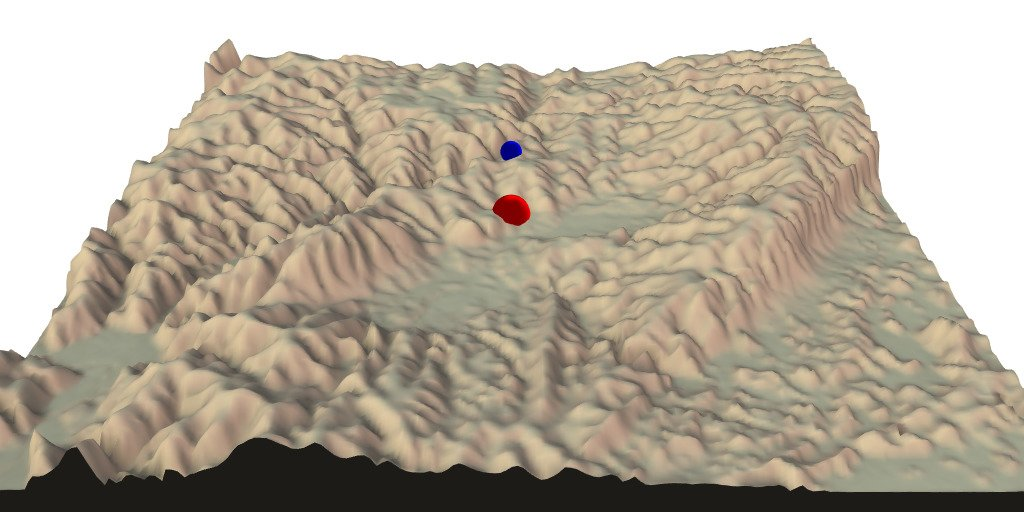{rayshader} is a fantastic package for 3D mapping, but it comes with the limitations of {rgl}. I always found it a shame not being able to travel in all directions over my 3D objects. In this blog post, I will show a trick to simulate particle trajectory in 3 dimensions like in a “first person” video game.

Functions presented here are available in package {maps2ray} on my Github: https://github.com/statnmap/maps2ray

## A few years earlier…

A few years ago, I was modelling flatfish larval dispersal in the Eastern Channel for my PhD thesis (Link to the thesis at the bottom of my CV). At that time, I dreamt I would have time to create a little video that would follow one larvae during its long journey to the coasts, then becoming a young flatfish… I never found time to do this. The only animation I could do was the following one. Symbol of the particle changes according to developmental stage of larvae. At some point, there are big enough to settle over a nursery, if reached.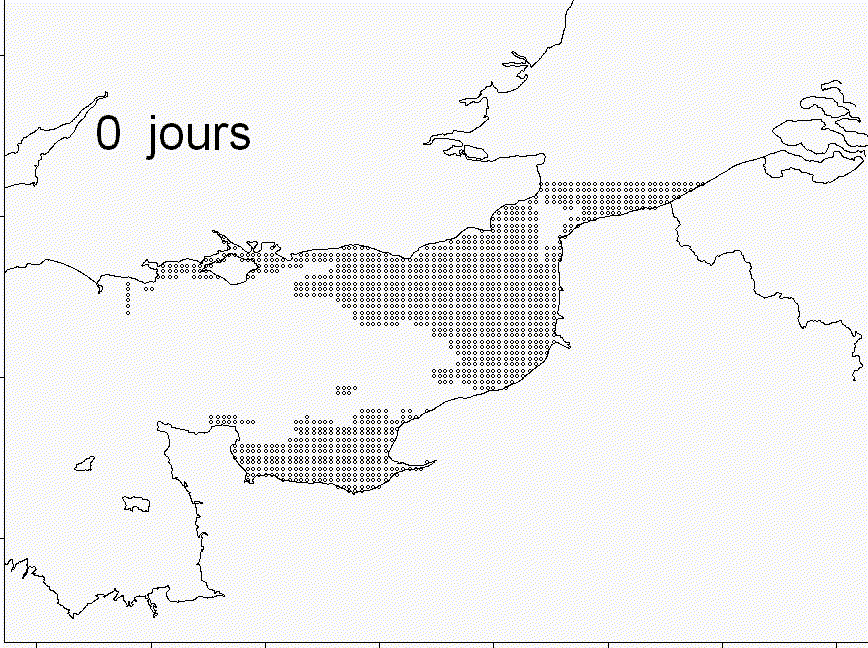Figure 1: Solea solea larval dispersal

Now, with {rayshader}, my dreams may become true !

## Packages

``````# devtools::install_github("tylermorganwall/rayshader")
# remotes::install_github("statnmap/mesh2ray")
library(sf)
library(raster)
library(rasterVis)
library(ggplot2)
library(dplyr)
library(rgl)``````

## Get an altitude map

This is the map of altitude of Department ‘Loire-atlantique’ in France, downloaded from IGN website.

``````# extraWD <- "." # Uncomment for the script to work
if (!file.exists(file.path(extraWD, "Alti_mosaic_L93.tif"))) {
}

altitude <- raster(file.path(extraWD, "Alti_mosaic_L93.tif")) # %>%
# aggregate(fact = 2)
plot(altitude)``````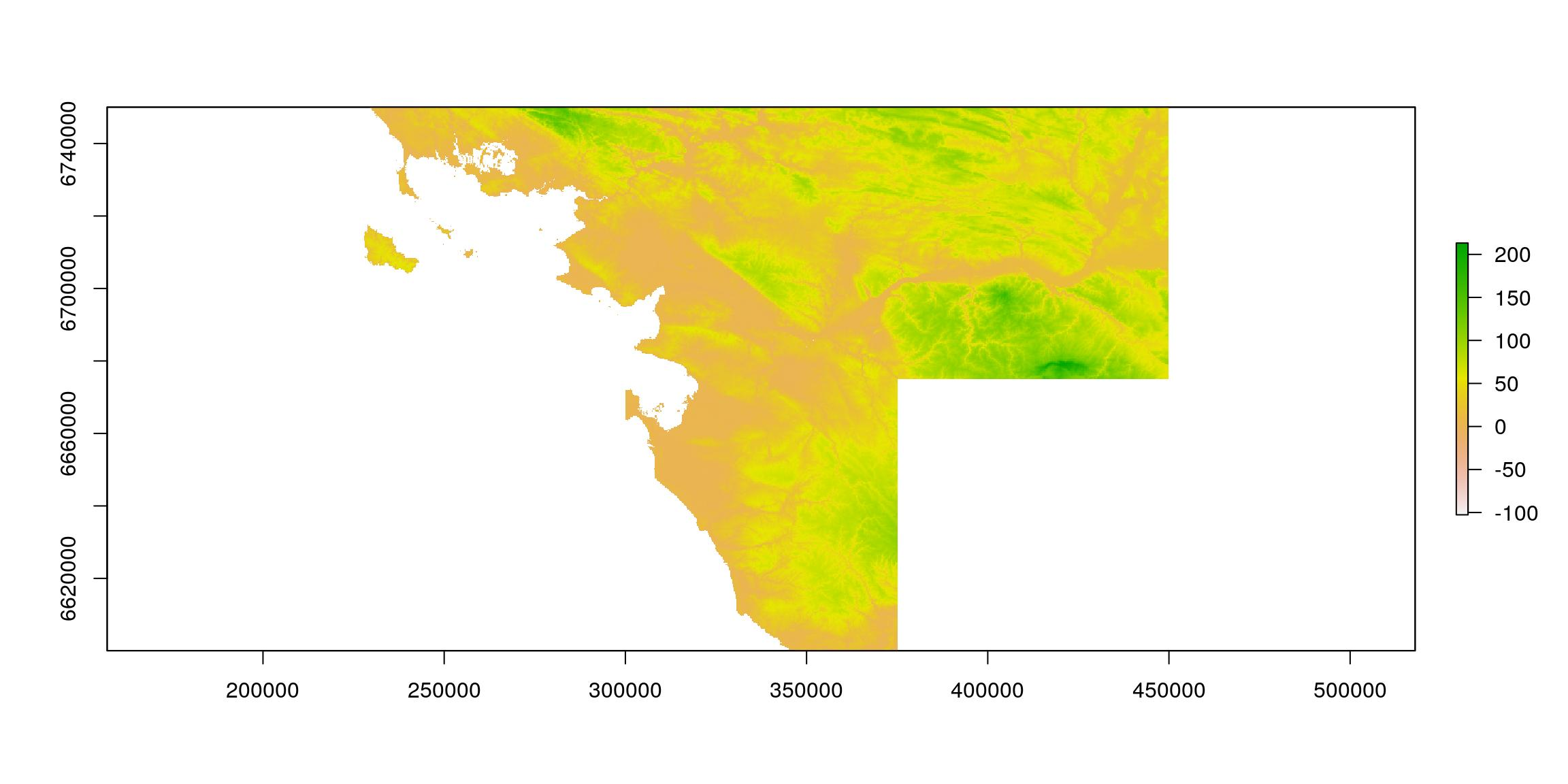Figure 2: Loire-atlantique Altitude

## Create a trajectory as {sf} spatial object

We first create a straight line that we sample regularly into points.

``````line_mat <- matrix(c(250000, 450000, 6720000, 6720000), ncol = 2)
line_traject <- st_linestring(x = line_mat)
point_traject <- st_sample(line_traject, size = 50, type = "regular") %>%
st_set_crs(2154) %>%
st_sf()

# Plot
gplot(altitude) +
geom_tile(aes(fill = value)) +
geom_sf(data = point_traject, colour = "white", inherit.aes = FALSE) +
scale_fill_viridis_c()``````Figure 3: Simple trajectory with {sf}

This is not a secret, I like to play with {rayshader}. Other articles of my own are here as my experiments with {rayshader}. Also, I like to play with spatial objects. For this, package {sf} is a good start.
Hence, in my talk called “Everything but maps with spatial tools” at SatRday Paris, I presented some 3D visualisations overlaid with {sf} spatial objects. I do not think I shared the script to realise this. So here it is.
You will find below a function that modifies {sf} objects to be overlaid on a {raster} object using {rayshader}: `sf_proj_as_ray()`. This recalculate positions in the future coordinates reference system of your raster transformed for rayshading.

This function is in package {maps2ray} on my Github: https://github.com/statnmap/maps2ray

``````#' Modify sf data to plot over rayshader
#'
#' @param r original raster used in rayshader
#' @param sf sf object to add on plot (with or without z column)
#' @param z_pos z-position of the sf on the 3d map if not in dataset
#' @param zscale if z-column existed in the dataset
#' @param as_sf Logical. Return sf object or not
#' @param groups Logical. Whether to group coordinates in blocks according to original structure.
#' @param crop Whether to crop sf object to extent of raster
#'
#' @export
sf_proj_as_ray <- function(r, sf, z_pos = 1, zscale = 10, as_sf = TRUE, groups = TRUE, crop = TRUE) {

# Crop to extent of raster
if (isTRUE(crop)) {
sf <- st_crop(sf, st_bbox(r))
}

if (!is.null(sf\$z)) {
sf <- sf %>%
mutate(
L2 = 1:n(),
Z_ray = z / zscale
)
} else {
sf <- sf %>%
mutate(
L2 = 1:n(),
Z_ray = z_pos / zscale
)
}

coords <- sf %>%
st_coordinates() %>%
as.data.frame() %>%
as_tibble() %>%
mutate(
X_ray = (X - xmin(r)) / xres(r),
# Y_ray = -1*(Y - ymin(r)) / yres(r)
Y_ray = 1*(Y - ymin(r)) / yres(r)
)

if (!"L1" %in% names(coords)) {
coords <- coords %>%
mutate(L1 = 1,
L2 = 1:n()
)
} else if (!"L2" %in% names(coords)) {
coords <- coords %>%
group_by(L1) %>%
mutate(L2 = L1,
L1b = 1:n()) %>%
ungroup() %>%
mutate(L1 = L1b) %>%
select(-L1b)
}

if (isTRUE(as_sf)) {
coords_sf <- coords %>%
select(-X, -Y) %>%
st_as_sf(coords = c("X_ray", "Y_ray")) %>%
group_by(L1, L2) %>%
summarise(do_union = FALSE) %>%
left_join(as.data.frame(sf) %>% select(-geometry))

if (all(st_is(sf, c("POLYGON", "MULTIPOLYGON")))) {
coords_sf <- coords_sf %>%
st_cast("POLYGON")
} else  if (all(st_is(sf, c("LINESTRING", "MULTILINESTRING")))) {
coords_sf <- coords_sf %>%
st_cast("LINESTRING")
}else if (all(st_is(sf, c("POINT", "MULTIPOINT")))) {
coords_sf <- coords_sf %>%
st_cast("POINT")
}
}
if (isTRUE(groups)) {
coords_grp <- coords %>%
mutate(Y_ray = -1 * Y_ray) %>%
left_join(st_drop_geometry(sf)) %>%
group_by(L1, L2) %>%
tidyr::nest(coords = c(X_ray, Z_ray, Y_ray))
}

if (isTRUE(as_sf) & isTRUE(groups)) {
res <- list(sf = coords_sf, coords = coords_grp)
} else if (isTRUE(as_sf) & !isTRUE(groups)) {
res <- list(sf = coords_sf, coords = coords)
} else if (isTRUE(as_sf) & !isTRUE(groups)) {
res <- list(coords = coords_grp)
} else {
res <- list(coords = coords)
}
return(res)
}``````

## Show points over raster in rayshader

Now, you can plot outputs of the function.

• Calculate {sf} object coordinates in future rayshader coordinates system using `sf_proj_as_ray()`
• Define `zscale <- 10` for all {rayshader} objects
``````zscale <- 5
points_ray <- sf_proj_as_ray(r = altitude, sf = point_traject,
z_pos = maxValue(altitude) + 10,
zscale = zscale)``````
• Create the {rayshader} terrain object to be used in both 2D and 3D outputs
``````# Transform raster as matrix
datamat <- t(as.matrix(altitude))
ambmat <- ambient_shade(datamat, zscale = zscale)
raymat <- ray_shade(datamat, zscale = zscale, lambert = TRUE)

# Create ray_image
ray_image <- datamat %>%
• Plot rayshader image in 2D and add points with new coordinates reference system of {rayshader}: `sf` list in the output of `sf_proj_as_ray()`.
• The red point is a target for the following paragraphs
``````# plot rayshader in 2D
plot_map(ray_image)
plot(points_ray\$sf, col = "blue", pch = 20, add = TRUE, reset = FALSE)
plot(points_ray\$sf[10,], col = "red", pch = 20, cex = 2, add = TRUE, reset = FALSE)``````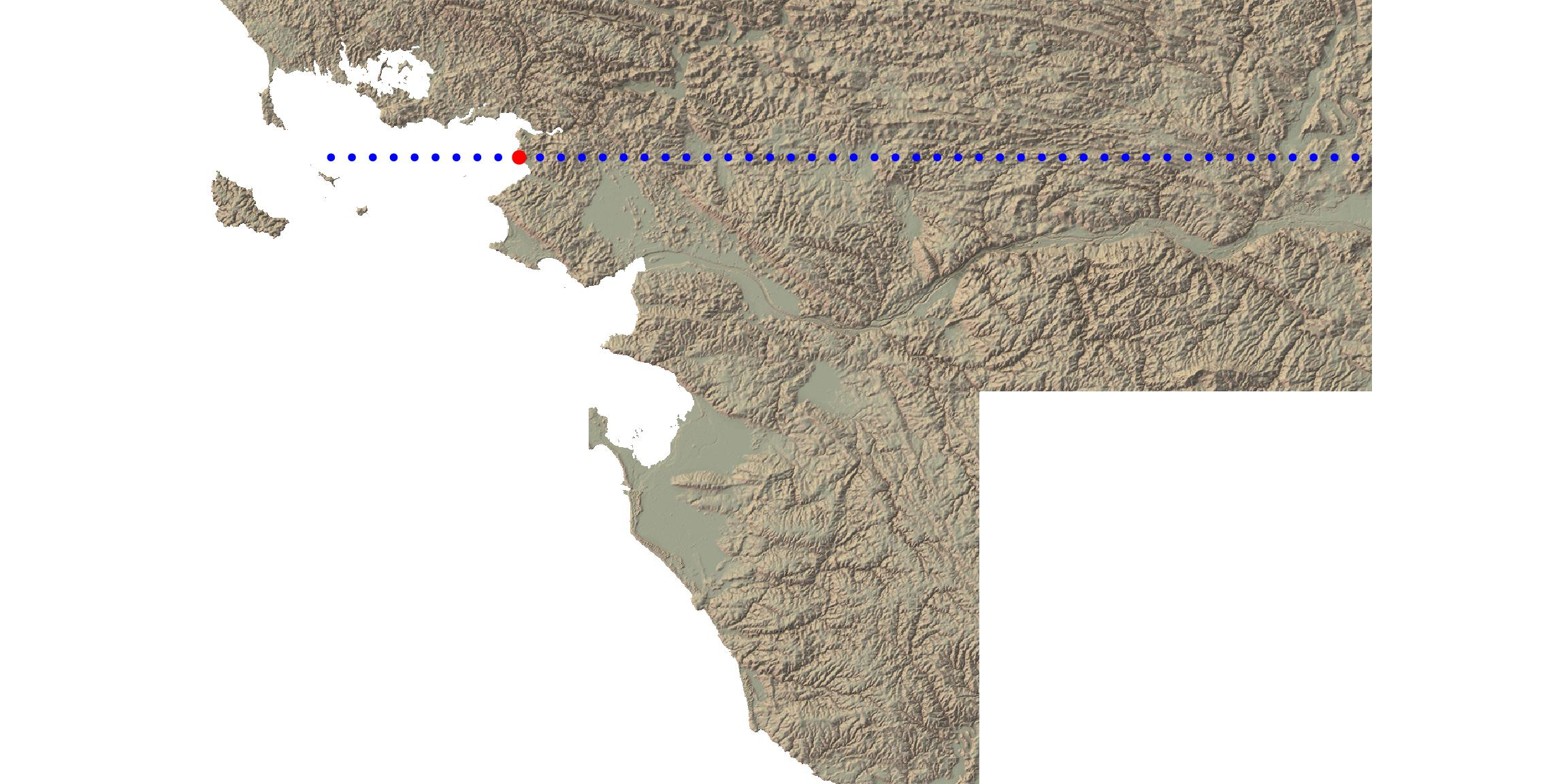Figure 4: Trajectory over {rayshader} in 2D

• Plot {rayshader} image in 3D and overlay points with the new coordinates reference system of {rayshader}: `coords` list in the output of `sf_proj_as_ray()`.
``````ray_image %>%
plot_3d(
datamat,
zscale = zscale, windowsize = c(500, 500),
soliddepth = -max(datamat, na.rm = TRUE)/zscale,
water = TRUE, wateralpha = 0,
theta = 15, phi = 40,
zoom = 0.6,
fov = 60)

spheres3d(
bind_rows(points_ray\$coords\$coords),
alpha = 1)

i <- 10
spheres3d(
points_ray\$coords\$coords[[i]],
alpha = 1)

rgl::snapshot3d(file.path(extraWD, "ray3dtotal.png"))
rgl::rgl.close()``````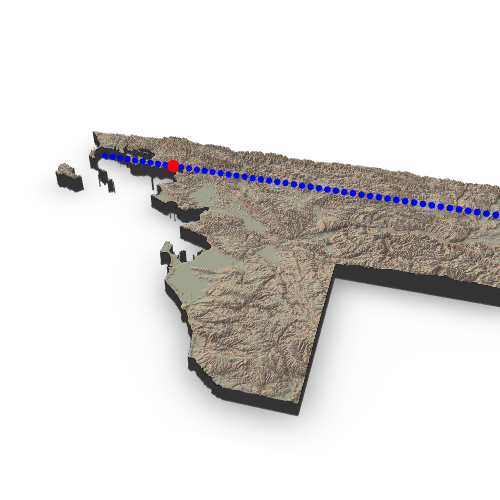Figure 5: Trajectory over {rayshader} in 3D

## Show a cropped areas

The raster is already rayshaded. If I want to zoom on a specific area, I do not want to calculate again the shading. I thus explored the reference system to crop matrices and recalculate point position on a smaller part of the raster.
To do so, I focus on one point of the trajectory (the red one above) and create a smaller window around this point.

• We can verify the correct cropping in 2D
``````# plot rayshader in 2D
dim(ray_image)
dim(datamat)
# Need a matrix without zero for render_label
data1 <- datamat
data1[is.na(data1)] <- 0

# Point as reference
i <- 10
position <- points_ray\$coords\$coords[[i]]
window <- 100
# Create a window around point
bounds <- tibble(
Xmin = max(round(position) - window, 0), # X
Xmax = min(round(position) + window, dim(datamat)),
Ymin_pos = max(round(position) - window, -dim(datamat)), # Y
Ymax_pos = min(round(position) + window, 0),
Ymin_row = dim(datamat) + Ymin_pos + 1,
Ymax_row = dim(datamat) + Ymax_pos + 1
)

# Height of the block (min - 1/5 of total height) to correct z
# soliddepth <- min(datamat, na.rm = TRUE))/zscale)
one_5 <- (max(datamat, na.rm = TRUE) - min(datamat, na.rm = TRUE))/5
soliddepth <- (min(datamat, na.rm = TRUE) - one_5)/zscale

# Calculate new position of the point on the cropped raster
position_bounds <- position %>%
mutate(
X_ray = X_ray - bounds\$Xmin + 1,
Y_2d = Y_ray - bounds\$Ymin_pos + 1,
Y_ray = -1 * (Y_ray - bounds\$Ymin_pos + 1),
Z_ray = Z_ray
)

# Verify cropped area and point position
plot_map(ray_image[bounds\$Ymin_row:bounds\$Ymax_row,bounds\$Xmin:bounds\$Xmax,])
points(position_bounds[,c("X_ray", "Y_2d")], col = "red", pch = 20, cex = 2)``````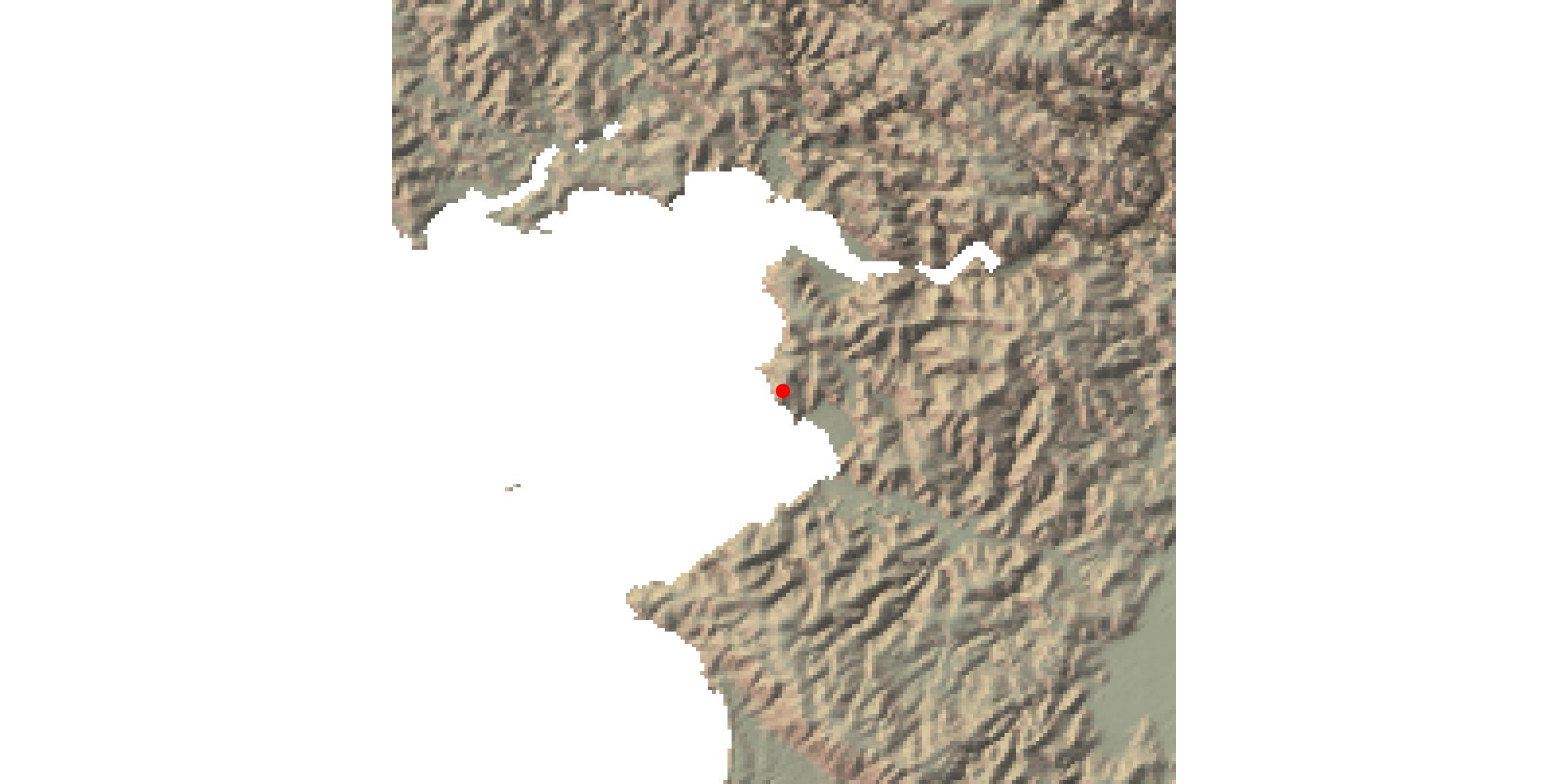Figure 6: Verify cropping area in {rayshader} 2D

• Then in 3D
``````ray_image[bounds\$Ymin_row:bounds\$Ymax_row,bounds\$Xmin:bounds\$Xmax,] %>%
plot_3d(
datamat[bounds\$Xmin:bounds\$Xmax, bounds\$Ymin_row:bounds\$Ymax_row],
zscale = zscale, windowsize = c(500, 500),
soliddepth = soliddepth,
water = TRUE, wateralpha = 0,
theta = -90, phi = 30,
zoom = 0.4,
fov = 80)

render_label(
data1[bounds\$Xmin:bounds\$Xmax, bounds\$Ymin_pos:bounds\$Ymax_pos],
x = pull(position_bounds[1, "X_ray"]),
y = -pull(position_bounds[1, "Y_ray"]),
z = 200,
zscale = zscale,
text = "Here",
color = "red"
)

spheres3d(position_bounds[,c("X_ray", "Z_ray", "Y_ray")],
color = "red", add = TRUE, lwd = 5, radius = 5,
alpha = 1)

rgl::snapshot3d(file.path(extraWD, "ray3dcrop.png"))
rgl::rgl.close()``````Figure 7: Trajectory over {rayshader} in 3D

## What about playing a film now ?

I can create the scenes around each point of the trajectory and follow this sphere over the scene in a gif.

• Let’s put everything together in a function

This function is in package {maps2ray} on my Github: https://github.com/statnmap/maps2ray

``````#' Create a cropped scene
#'
#' @param datamat Original raster transformed as matrix with t(as.matrix(raster))
#' @param position dataframe of point coordinates in the {rayshader} system
#' as created using sf_proj_as_ray()
#' @param position_next dataframe of another point coordinates in the {rayshader}
#'system as created using sf_proj_as_ray()
#' @param window size of the square window in pixels around the position
#' @param zscale Ratio between Z and x/y. Adjust the zscale down to exaggerate
#' elevation features. Better to use the same for the entire workflow.
#' @param zoom Zoom factor. Reduce to zoom in.
#' @param windowsize Size of the rgl window
render_cropped_scene <- function(ray_image, datamat, position,
position_next,
window = 100,
zscale = 5, zoom = 0.4,
windowsize = c(500, 500)) {
# Create a window around point
bounds <- tibble(
Xmin = max(round(position) - window, 0), # X
Xmax = min(round(position) + window, dim(datamat)),
Ymin_pos = max(round(position) - window, -dim(datamat)), # Y
Ymax_pos = min(round(position) + window, 0),
Ymin_row = dim(datamat) + Ymin_pos + 1,
Ymax_row = dim(datamat) + Ymax_pos + 1
)

# Height of the block (min - 1/5 of total height) to correct z
# soliddepth <- min(datamat, na.rm = TRUE))/zscale)
one_5 <- (max(datamat, na.rm = TRUE) - min(datamat, na.rm = TRUE))/5
soliddepth <- (min(datamat, na.rm = TRUE) - one_5)/zscale

# Calculate new position of the point on the cropped raster
position_bounds <- position %>%
mutate(
X_ray = X_ray - bounds\$Xmin + 1,
Y_2d = Y_ray - bounds\$Ymin_pos + 1,
Y_ray = -1 * (Y_ray - bounds\$Ymin_pos + 1),
Z_ray = Z_ray #/zscale
)

# Plot cropped 3D output
ray_image[bounds\$Ymin_row:bounds\$Ymax_row,bounds\$Xmin:bounds\$Xmax,] %>%
plot_3d(
datamat[bounds\$Xmin:bounds\$Xmax, bounds\$Ymin_row:bounds\$Ymax_row],
zscale = zscale, windowsize = windowsize,
# soliddepth = -min(datamat, na.rm = TRUE)/zscale,
soliddepth = soliddepth,
water = TRUE, wateralpha = 0,
theta = -90, phi = 30,
zoom = zoom,
fov = 80)

spheres3d(position_bounds[,c("X_ray", "Z_ray", "Y_ray")],
color = "red", add = TRUE, lwd = 5, radius = 5,
alpha = 1)

if (!missing(position_next)) {
position_next_bounds <- position_next %>%
mutate(
X_ray = X_ray - bounds\$Xmin + 1,
Y_2d = Y_ray - bounds\$Ymin_pos + 1,
Y_ray = -1 * (Y_ray - bounds\$Ymin_pos + 1),
Z_ray = Z_ray
)

spheres3d(position_next_bounds[,c("X_ray", "Z_ray", "Y_ray")],
color = "blue", add = TRUE, lwd = 5, radius = 3,
alpha = 1)
}
}``````
• Create a film using all positions of the trajectory
``````path_gif <- file.path(extraWD, "film_follow_trajectory.gif")
if (!file.exists(path_gif)) {

# Number of frame = Number of points
n_frames <- length(points_ray\$coords\$coords)
savedir <- file.path(extraWD, "film_follow_trajectory")
if (!dir.exists(savedir)) {
dir.create(savedir, showWarnings = FALSE)
}
img_frames <- file.path(
savedir,
paste0("film_", formatC(seq_len(n_frames), width = 3, flag = "0"), ".png")
)

# create all frames
for (i in seq_len(n_frames)) {
# i <- 20
position <- points_ray\$coords\$coords[[i]]
if (i < n_frames) {
position_next <- points_ray\$coords\$coords[[i + 1]]
render_cropped_scene(ray_image, datamat, position,
position_next)
} else {
# Last one
render_cropped_scene(ray_image, datamat, position)
}
# Save img
rgl::snapshot3d(img_frames[i])
rgl::clear3d()
}
rgl::rgl.close()

# Create gif
path = path_gif,
delay = 6/n_frames)
}``````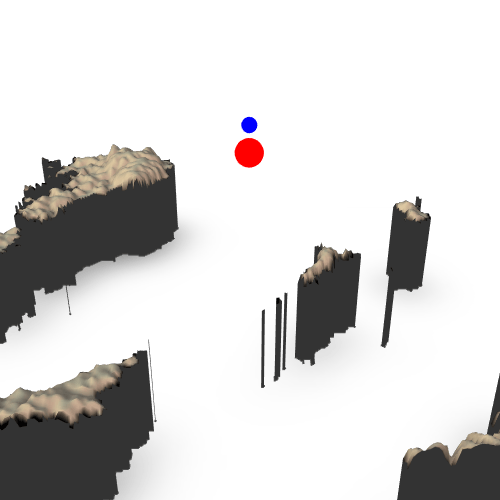Figure 8: Film of trajectory over {rayshader} in 3D

## Add points with real altitudes

• Extract altitudes at points position to be used in the film
• Recalculate positions with the {rayshader} coordinates reference system
• Build the film !
``````# Extract z
point_traject_z <- point_traject %>%
st_cast("POINT") %>%
mutate(z = raster::extract(altitude, point_traject %>% st_cast("POINT")),
z = ifelse(is.na(z), 4, z + 1))

points_ray_z <- sf_proj_as_ray(r = altitude, sf = point_traject_z,
zscale = zscale)

# Create film
path_gif_alt <- file.path(extraWD, "film_follow_trajectory_altitude.gif")
if (!file.exists(path_gif_alt)) {

# Number of frame = Number of points
n_frames <- length(points_ray_z\$coords\$coords)
savedir <- file.path(extraWD, "film_follow_trajectory_altitude")
if (!dir.exists(savedir)) {
dir.create(savedir, showWarnings = FALSE)
}
img_frames <- file.path(
savedir,
paste0("film_", formatC(seq_len(n_frames), width = 3, flag = "0"), ".png")
)

# create all frames
for (i in seq_len(n_frames)) {
# i <- 20
position <- points_ray_z\$coords\$coords[[i]]
if (i < n_frames) {
position_next <- points_ray_z\$coords\$coords[[i + 1]]
render_cropped_scene(ray_image, datamat, position,
position_next)
} else {
# Last one
render_cropped_scene(ray_image, datamat, position)
}
# Save img
rgl::snapshot3d(img_frames[i])
rgl::clear3d()
}
rgl::rgl.close()

# Create gif
path = path_gif_alt,
delay = 6/n_frames)
}``````Figure 9: Film of trajectory over {rayshader} in 3D

## Go further

Find the script of this blog post on my github: https://github.com/statnmap/blog_tips
Functions presented here are available in package {maps2ray} on my Github: https://github.com/statnmap/maps2ray

Now, I am waiting for other people to transform this as a video game in a Shiny Application !

``````# For header
i <- 20
position <- points_ray_z\$coords\$coords[[i]]
position_next <- points_ray_z\$coords\$coords[[i + 1]]
render_cropped_scene(ray_image, datamat, position,
position_next, windowsize = c(1024, 512),
zoom = 0.3)

###### Citation:

``` Rochette Sébastien. (2019, Oct. 06). "Follow moving particle trajectory over raster with {rayshader}". Retrieved from https://statnmap.com/2019-10-06-follow-moving-particle-trajectory-on-raster-with-rayshader/. ```
``````@misc{Roche2019Follo,